## 2. 6. 3 Geometric Examples

In some cases it is necessary to determine the volume of a cell. For instance, many formulae for distinct integrals lead to a formulation which contains the original volume. In the following case the calculation of the volume is shown for a tetrahedron. A typical formulation of the volume of a general simplex can be defined as follows.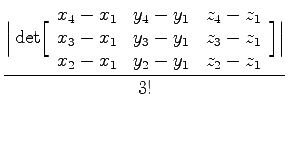(2.60)

For the determination of this determinant value it is necessary to obtain one definite vertex from the set of incident vertices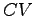. This can be easily provided by a first vertex function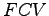which returns only one vertex of the cell. Furthermore, it is necessary to remove the respective vertex from the set of vertices. Using the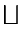operator, a matrix can be provided. The vector value quantity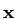contains the coordinate of the vertices.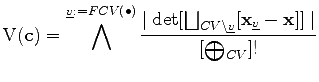(2.61)

The expression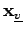evaluates the coordinate of the first of the incident vertices passed by thefunction. The accumulation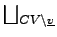forms a vector in which the resulting vectors of the subtractions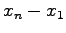are inserted.

Michael 2008-01-16